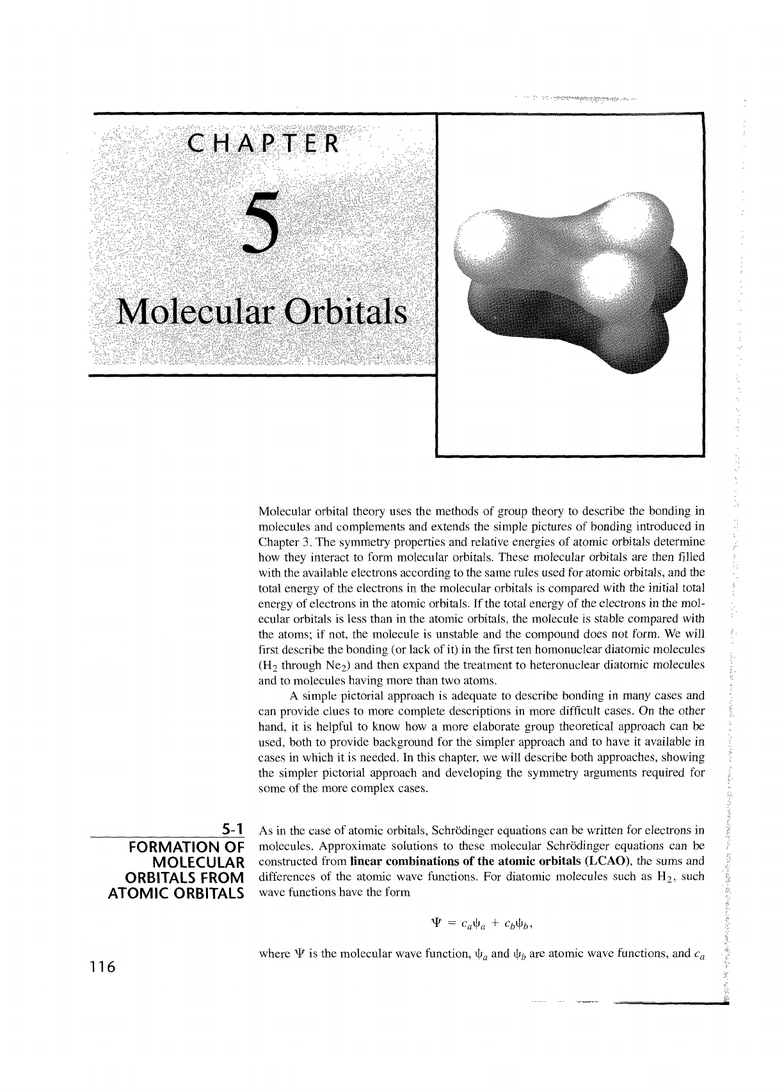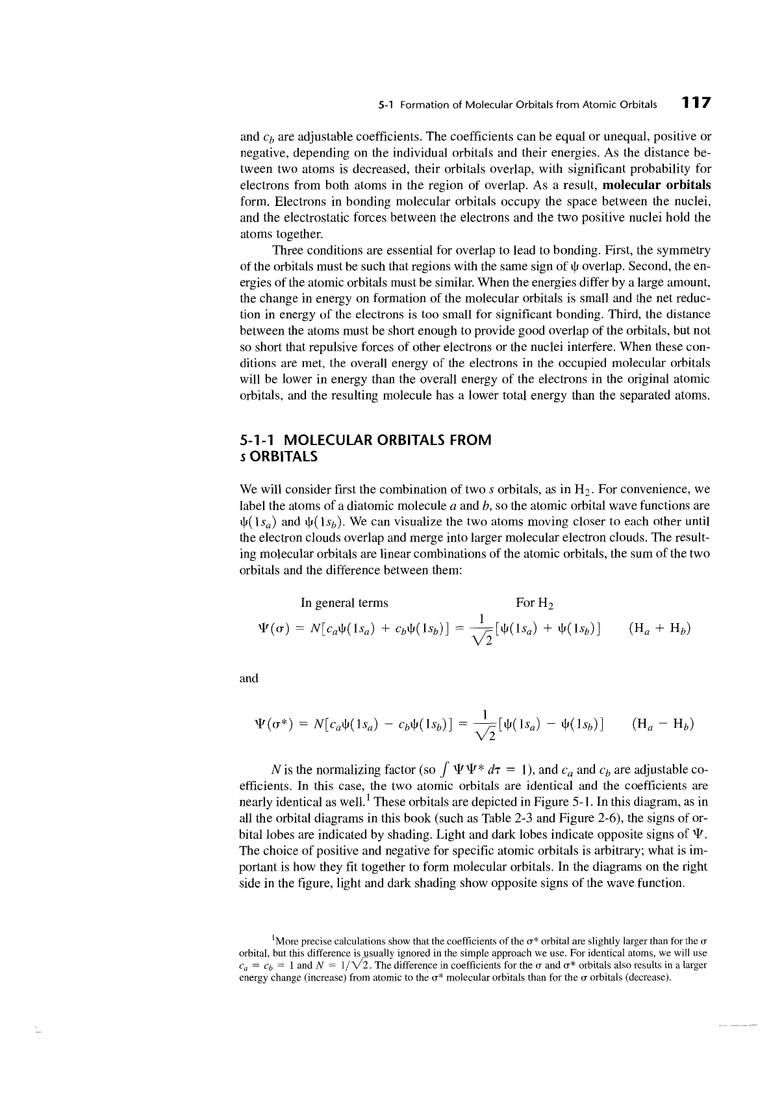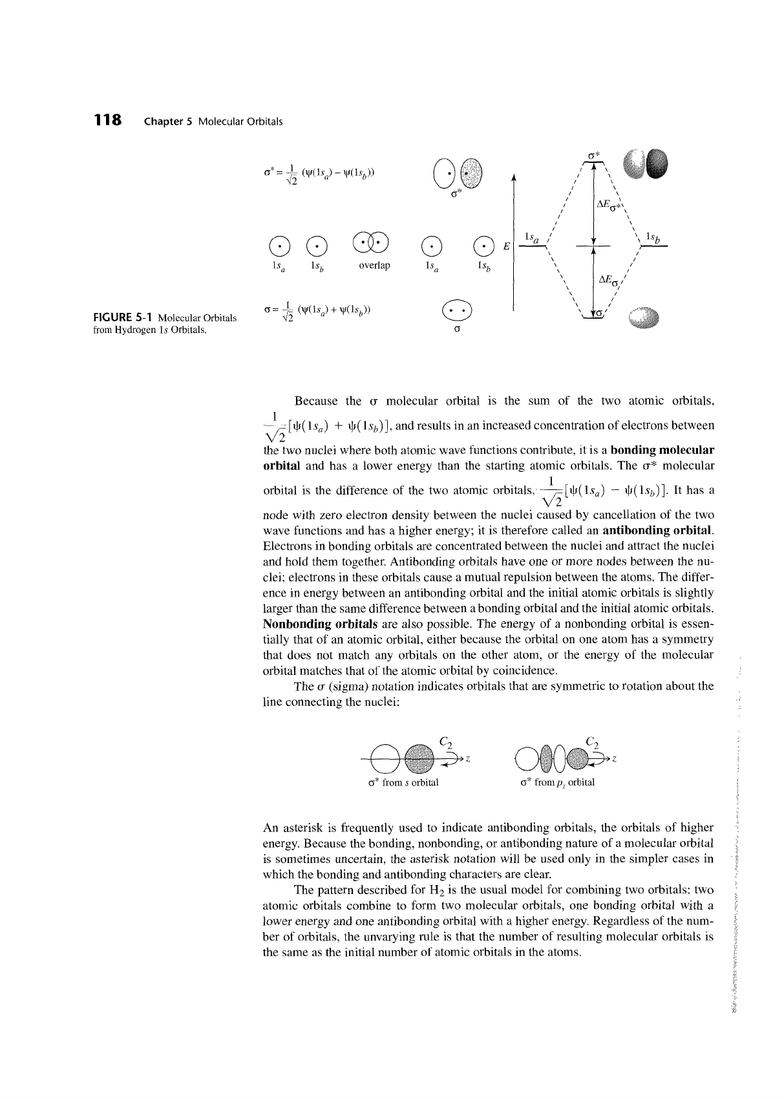# CHEM 212 Lecture Notes - Noble Gas, Tral, Bond Order

51 views45 pages
School
McGill University
Department
Chemistry
Course
CHEM 212Molecular orbital theory uses the methods of group theory to describe the bonding in
inolecules and
complements
and extends the simple pictures of bonding introduced in
Chapter
3.
The symmetry properties and relative energies of atomic orbitals determine
how they interact to form molecular orbitals. These molecular orbitals
are
then filled
with the available electrons according to the same rules used for atomic orbitals, and the
total energy of the electrons in the molecular orbitals is compared with the initial total
energy of electrons in the atomic orbitals. If the total energy of the electrons in the mol-
ecular orbitals is less than in the atomic orbitals, the molecule is stable compared with
the atoms; if not, the molecule is unstable and the compound does not form. We will
first describe the bonding (or lack of it) in the first ten homonuclear diatomic molecules
(H2
through Ne2) and then expand the treatment to heteronuclear diatomic molecules
and to molecules having more than two atoms.
A
simple pictorial approach is adequate to describe bonding in many cases and
can provide clues to more complete descriptions in more difficult cases. On the other
hand, it is helpful to know how a more elaborate group theoretical approach can
be
used, both to provide background for the simpler approach and to have it available in
cases in which it is needed. In this chapter, we will describe both approaches, showing
the simpler pictorial approach and developing the symmetry arguments required for
some of the more complex cases.
5-1
As in the case of atomic orbitals, Schrodinger equations can he written for electrons in
FORMATION OF
molecules. Approximate solutions to these molecular Schrodinger equations can be
MOLECULAR
constructed from
linear combinations of the atomic orbitals
(LCAO),
the sums and
ORBITALS FROM
differences of the atomic wave functions. For diatomic molecules such as Hz. such
ATOMIC ORBITALS
wave functions have the form
where
T
is the molecular wave function,
+,
and
\$h
are atomic wave functions, and
c,
Unlock document

This preview shows pages 1-3 of the document.
Unlock all 45 pages and 3 million more documents.5-1
Formation of Molecular Orbitals from Atomic Orbitals
1
17
and
cb
are adjustable coefficients. The coefficients can be equal or unequal, positive or
negative, depending on the individual orbitals and their energies. As the distance be-
tween two atoms is decreased, their orbitals overlap, wilh significant probability for
electrons from both atoms in the region of overlap. As a result,
molecular orbitals
form. Electrons in bonding molecular orbitals occupy the space between the nuclei,
and the electrostatic forces between the electrons and the two positive nuclei hold the
atoms together.
Three conditions are essential for overlap to lead to bonding. First, the symmetry
of the orbitals must be such that regions with the same sign of
+
overlap. Second, the en-
ergies of the atomic orbitals must be similar. When the energies differ by a large amount,
the change in energy on formation of the molecular orbitals is small and the net reduc-
tion in energy of the electrons is too small for significant bonding. Third, the distance
between the atoms must be short enough to provide good overlap of the orbitals, but not
so short that repulsive forces of other electrons or the nuclei interfere. When these con-
ditions are met, the overall energy of the electrons in the occupied molecular orbitals
will be lower in energy than the overall energy of the electrons in the original atomic
orbitals, and the resulting molecule has a lower total energy than the separated atoms.
5-1
-1
MOLECULAR ORBITALS FROM
s
ORBITALS
We will consider first the combination of two
s
orbitals, as in
Hz.
For convenience, we
label the atoms of a diatomic molecule
a
and
b,
so the atomic orbital wave functions are
+(lsa) and +(lsb). We can visualize the two atoms moving closer to each other until
the electron clouds overlap and merge into larger molecular electron clouds. The result-
ing molecular orbitals are linear combinations of the atomic orbitals, the sum of the two
orbitals and the difference between them:
In general terms For
H2
and
N
is the normalizing factor (so
T?lr*
d~
=
I),
and
c,
and
cb
efficients. In this case, the two atomic orbitals are identical and the coefficients are
nearly identical as well.' These orbitals are depicted in Figure
5-
1. In this diagram, as in
all the orbital diagrams in this book (such as Table
2-3
and Figure
2-6),
the signs of or-
bital lobes are indicated by shading. Light and dark lobes indicate opposite signs of
*.
The choice of positive and negative for specific atomic orbitals is arbitrary; what is im-
portant is how they fit together to form molecular orbitals. In the diagrams on the right
side in the figure, light and dark shading show opposite signs of the wave function.
ore
precise calculations show that the coefficients of the
a*
orbital are slightly larger than for the
a
orbital, but this difference is usually ignored in the simple approach we use. For identical atoms, we will use
c,
=
cb
=
1
and
N
=
I
/d.
The difference in coefficients for the
a
and
a*
orbitals also results in a larger
energy change (increase) from atomic to the
a*
molecular orbitals than for the
a
orbitals (decrease).
Unlock document

This preview shows pages 1-3 of the document.
Unlock all 45 pages and 3 million more documents.1
18
Chapter 5
Molecular Orbitals
Because the
u
molecular orbital is the sum of the two atomic orbitals,
1
-'
[\$(Is,)
+
\$(l
s~)], and results in an increased concentration of electrons between
6
the two nuclei where both atomic wave functions contribute, it is a
bonding molecular
orbital
and has a lower energy than the starting atomic orbilals. The
u*
molecular
1
orbital is the difference of the two atomic orbitals, ----[+(ls,)
-
+(lsb)]. It has a
di
node with zero electron density between the nuclei caused by cancellation of the two
wave functions and has a higher energy; it is therefore called an
antibonding orbital.
Electrons in bonding orbitals are concentrated between the nuclei and attract the nuclei
and hold them together. Antibonding orbitals have one or more nodes between the nu-
clei; electrons in these orbitals cause a mutual repulsion between the atoms. The differ-
ence in energy between an antibonding orbital and the initial atomic orbitals is slightly
larger than the same difference between a bonding orbital and the initial atomic orbitals.
Nonbonding orbitals
are also possible. The energy of a nonbonding orbital is essen-
tially that of an atomic orbital, either because the orbital on one atom has a symmetry
that does not match any orbitals on the other atom, or the energy of the molecular
orbital matches that of the atomic orbital by coincidence.
The u (sigma) notation indicates orbitals that are symmetric to rotation about the
line connecting the nuclei:
o"
from
s
orbital
o"
from
p,
orbital
An asterisk is frequently used to indicate antibonding orbitals, the orbitals of higher
.:
energy. Because the bonding, nonbonding, or antibonding nature of a molecular orbital
is sometimes uncertain, the asterisk notation will be used only in the simpler cases in
which the bonding and antibonding characters are clear.
The pattern described for
H2
is the usual model for combining two orbitals: two
atomic orbitals combine to form two molecular orbitals, one bonding orbital with a
lower energy and one antibonding orbital with a higher energy. Regardless of the num-
i
ber of orbitals, the unvarying rule is that the number of resulting molecular orbitals is
:i
the same as the initial number of atomic orbitals in the atoms.
Unlock document

This preview shows pages 1-3 of the document.
Unlock all 45 pages and 3 million more documents.